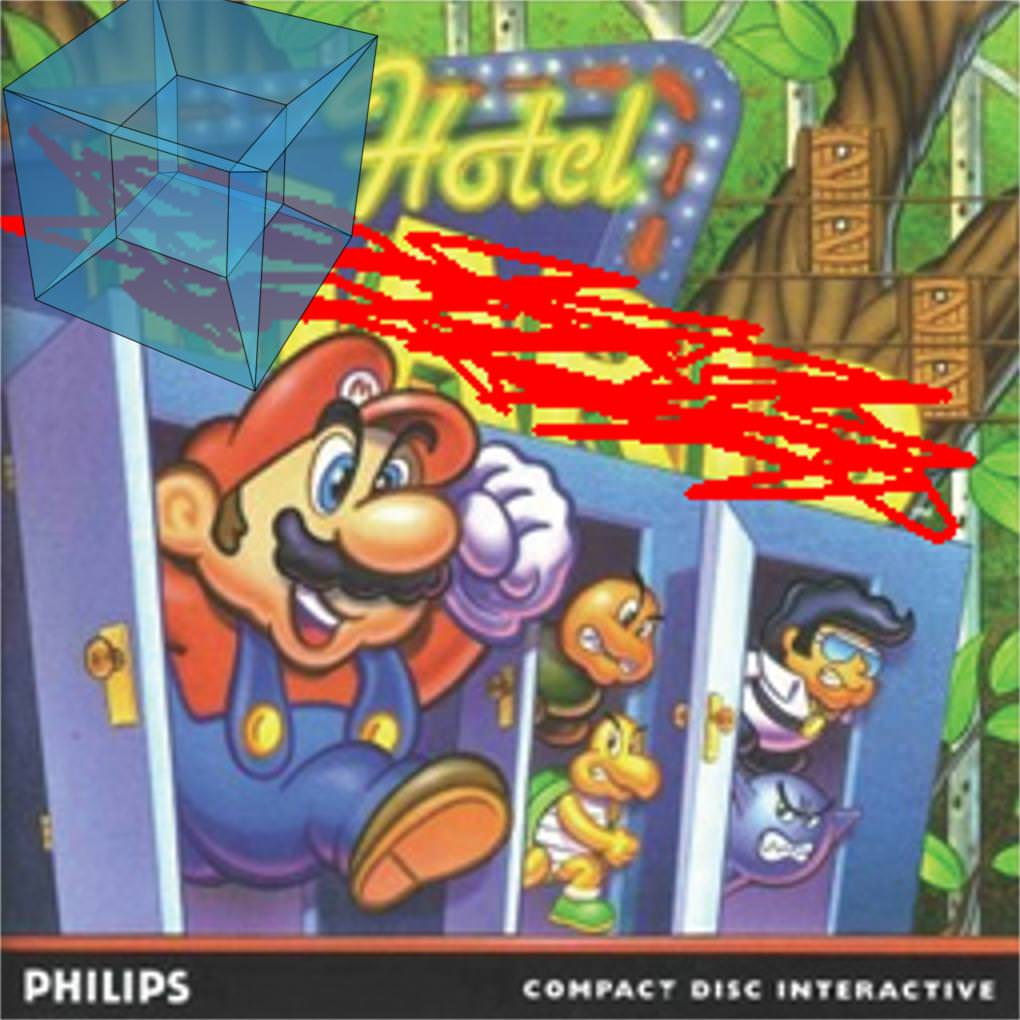## TLE '17 Contest 3 P5 - Hypercube Hotel

View as PDF

Points: 17 (partial)
Time limit: 1.0s
Java 2.0s
Python 2.0s
Memory limit: 256M

Author:
Problem types
Allowed languages
Ada, Assembly, Awk, Brain****, C, C#, C++, COBOL, CommonLisp, D, Dart, F#, Forth, Fortran, Go, Groovy, Haskell, Intercal, Java, JS, Kotlin, Lisp, Lua, Nim, ObjC, OCaml, Octave, Pascal, Perl, PHP, Pike, Prolog, Python, Racket, Ruby, Rust, Scala, Scheme, Sed, Swift, TCL, Text, Turing, VB, ZigThe Hypercube Hotel game is also very popular and universally acclaimed. Get it for the Phillips CD-i while it lasts!

The Hypercube Hotel is a strange new mathematically themed hotel, a competitor to Hilbert's Hotel. The hotel as an unusual way of numbering its rooms. Each room has andigit number so that the-th digit (from the left) is between 1 and. Conversely, every number in this form has a corresponding room. Room numbers are unique.

Two different rooms are called neighbours if for every, the-th digit of the two room numbers differ by at most 1. For example, the rooms with numbers 213 and 222 are neighbours but the rooms with numbers 111 and 131 are not neighbours. A room is not a neighbour of itself.

All the rooms in the hotel are occupied, so each room generates a certain amount of noise. If a room has numbercall this quantity. For each room, the noise level of that room is the sum of the amounts of noise generated by its neighbours. Given the amount of noise generated by each room, find the noise level of each room.

#### Constraints152535415570None

#### Input Specification

The first line contains the integer.

The next line containsintegers.

The nextlines each contain a single integer. The lines are given in increasing order of the room number.

#### Output Specification

For each room, output a line containing a single integer, the noise level of that room. Do this in increasing order of room number.

#### Sample Input

2
2 3
1
2
3
4
5
6

#### Sample Output

11
19
13
8
16
10

Java can pass. Mem complexity isand time complexity is